## RD Sharma Class 8 Solutions Chapter 9 Linear Equations in One Variable Ex 9.3

These Solutions are part of RD Sharma Class 8 Solutions. Here we have given RD Sharma Class 8 Solutions Linear Equations in One Variable Ex 9.3

Other Exercises

Question 1.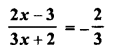Solution: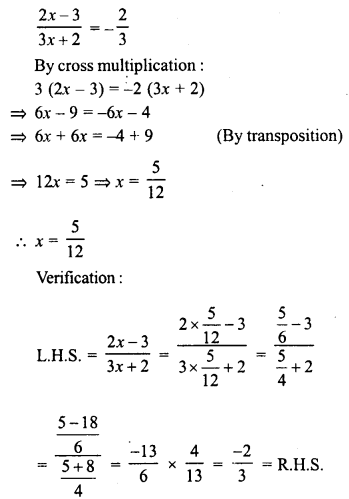Question 2.Solution: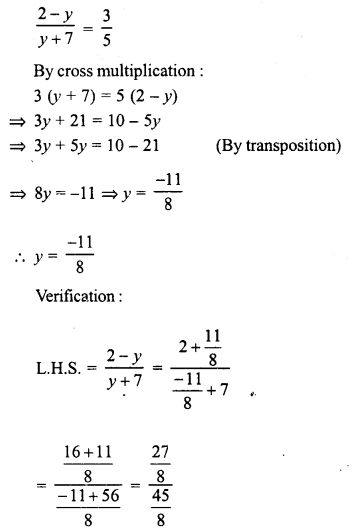Question 3.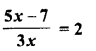Solution:Question 4.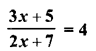Solution: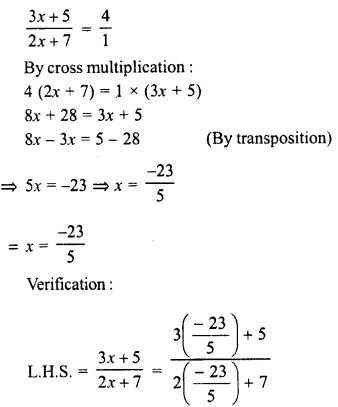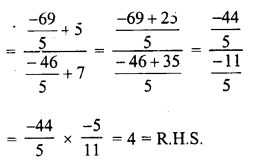Question 5.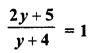Solution: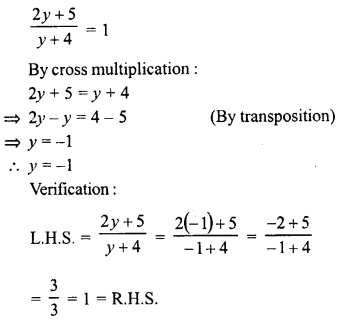Question 6.Solution: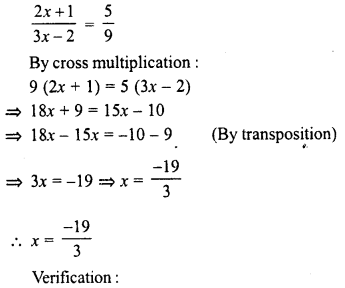Question 7.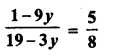Solution: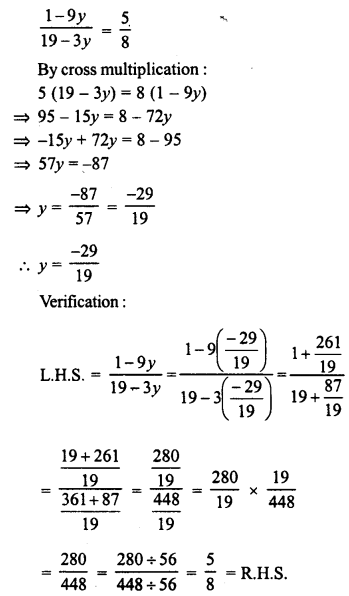Question 8.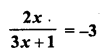Solution: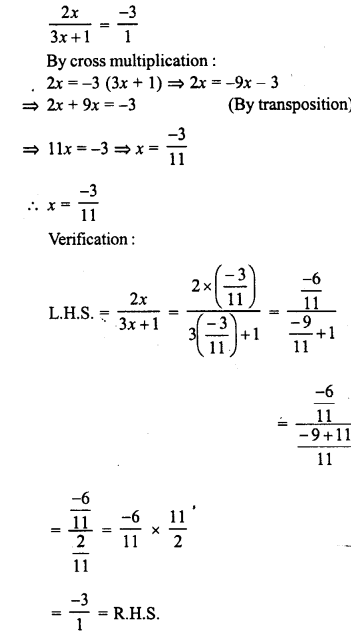Question 9.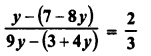Solution:Question 10.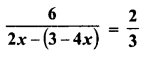Solution: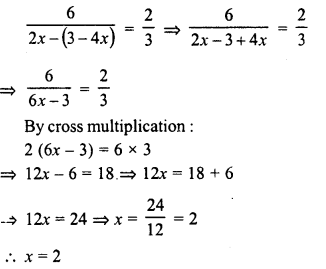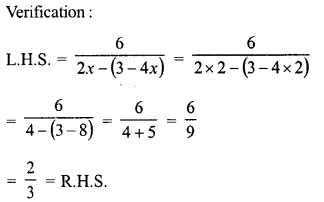Question 11.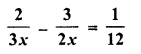Solution: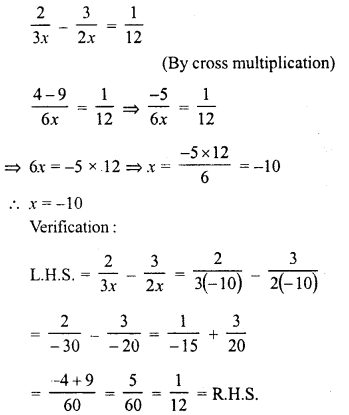Question 12.Solution: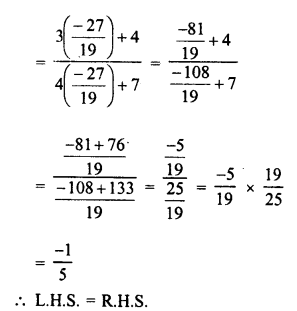Question 13.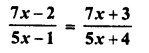Solution: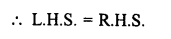Question 14.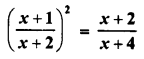Solution: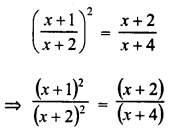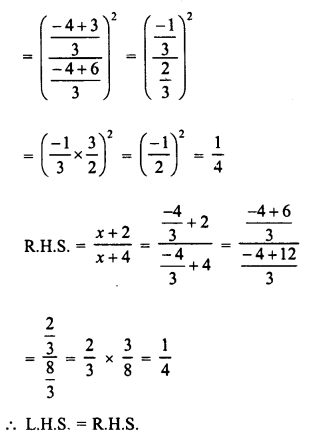Question 15.Solution: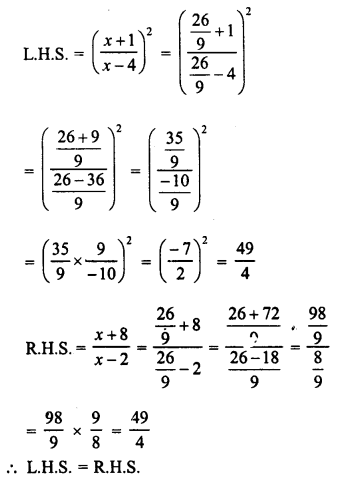Question 16.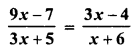Solution: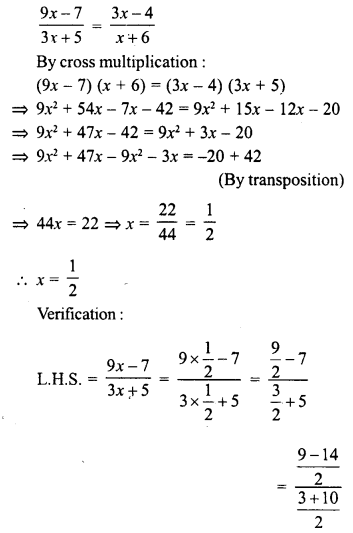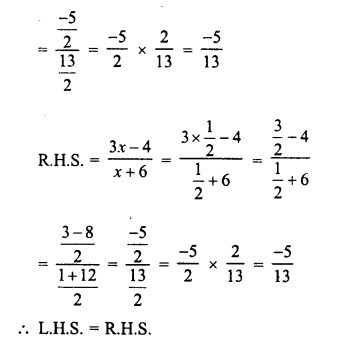Question 17.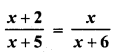Solution: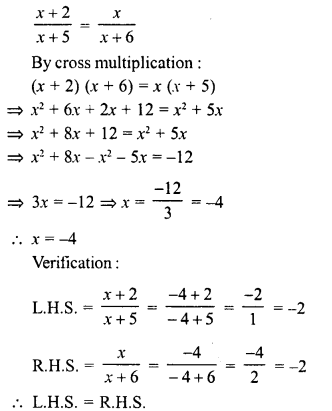Question 18.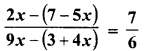Solution: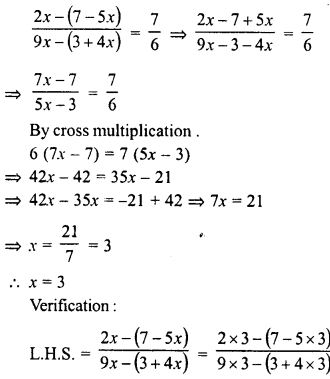Question 19.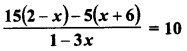Solution: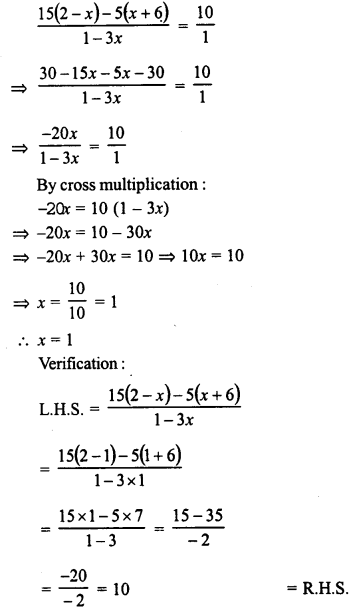Question 20.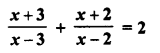Solution: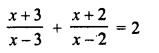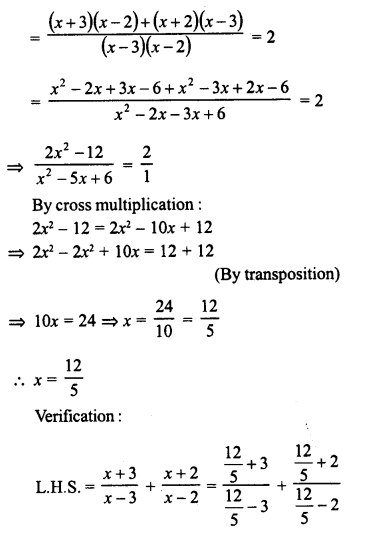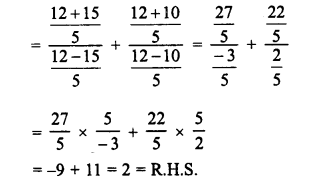Question 21.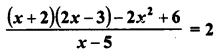Solution: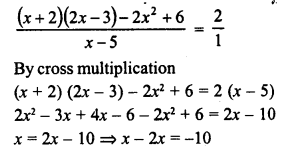Question 22.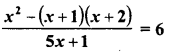Solution: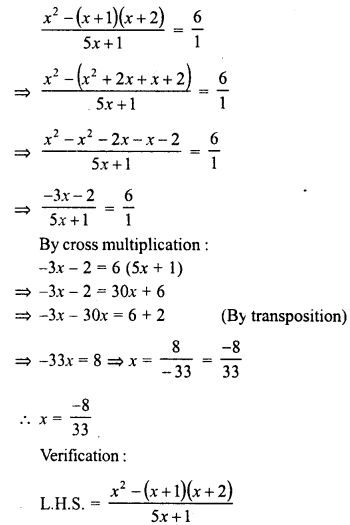Question 23.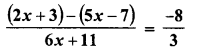Solution:Question 24.
Find a positive value of x for which the given equation is satisfied.Solution: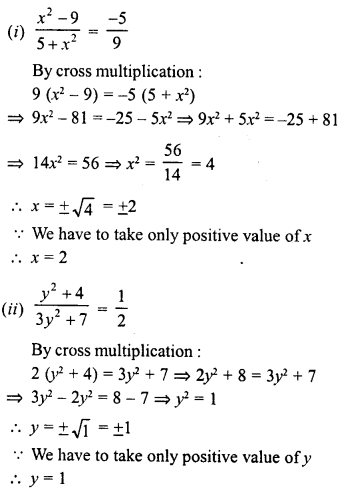Hope given RD Sharma Class 8 Solutions Linear Equations in One Variable Ex 9.3 are helpful to complete your math homework.

If you have any doubts, please comment below. Learn Insta try to provide online math tutoring for you.